Company Analysis

# 2021Q1: Limping Numbers, Slow Start For New Union Bank Plc Management

Rating: Hold
Current Market Price: N5.65

Loan to Debt Ratio: 63.54%
Year High: N6.10
Year Low: N4.50
Fair Value: N3.78
Equity Analyst:  Tunde Segun Jeariogbe

Introduction

• This report observes the first quarter financial numbers released for the period ended 30thMarch, 2021. We have compared the result with figures presented for the similar quarter of 2020 to establish growth, make projections, as well as valuation analysis.
• Generally, there was a drop in the top-line, and a marginal growth in bottom-line.
• We note the strong decline in Total Comprehensive Income for the period compared to figure stated in the comparable period of 2020.
• This drop resulted from the Fair value losses incurred on financial assets through the first three month of the year.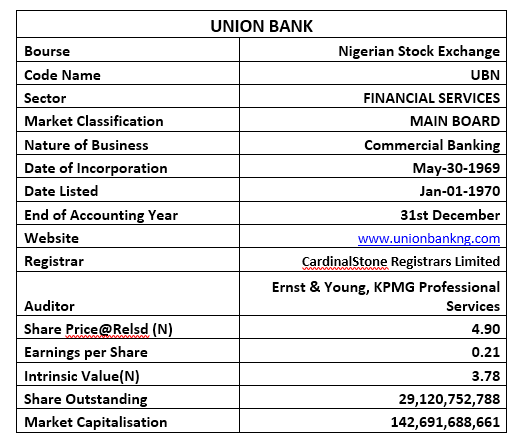Sources: Company Report, NGX, Investdata Research

Company figures

• Gross Earnings for the period is estimated at N36.793 billion, 16.21% below the N43.9909 billion reported at the end of 2020 first quarter.
• Interest Income dipped by 25.29% to N22.24 billion, as against the N29.78 billion reported in the corresponding period of last year.
• Interest Expenses is valued at N11.25 billion, a 24.10% downward adjustment from the N14.82 billion stated in its 2020Q1 numbers.
• Net Interest Income, therefore, dipped by 26.46% to N10.99 billion, versus N14.95 billion in the corresponding quarter.
• Operating Expenses was curtailed by a marginal 5.93% below the corresponding quarter as it is currently estimated at N15.42 billion, against N16.40 billion in the comparable quarter of 2020.
• Profit before Taxation is valued at N6.46 billion, 2.57% above the N6.29 billion achieved at the end of 2020 first quarter.
• Having accounted for tax expenses for the year, the management of Union Bank Plc reported N6.20 billion as Profit for the period, representing 3.19% above the corresponding quarter’s profit.
• As noted above, Total Comprehensive Income dipped by 58.80% to N843 million, versus N2.04 billion in the corresponding quarter.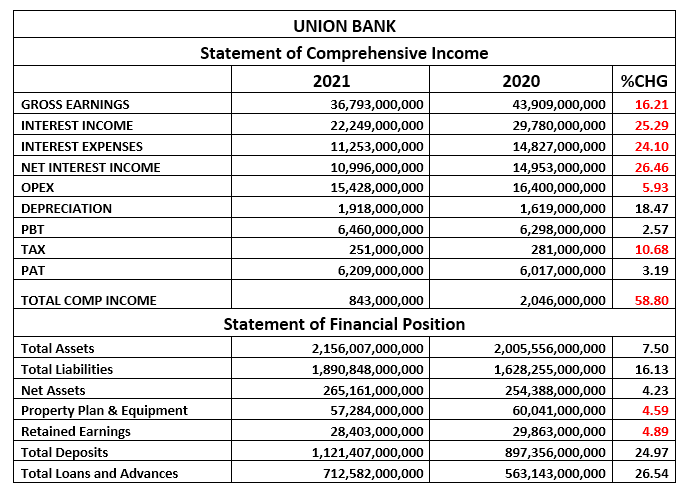Sources: Company Report, NGX, Investdata Research
• Total Assets valuation for the period stood at N2.15 trillion, 7.50% higher than the estimate at the end of 2020 first quarter.
• Total Liabilities on the other hand grew by 16.13% to N1.89 trillion versus N1.62 trillion in similar quarter of last year.
• Net Assets appreciated above the comparable quarter’s estimate by a marginal 4.23% to N265.16 billion, from N254.38 billion in 2020.
• Retained Earnings dipped by marginal 4.89% at N28.40 billion, versus N29.86 billion in the similar quarter of 2020.
• Total Customers Deposits at the end of 2020 first quarter business session improved by 24.97% to N1.12 trillion, as against N897.35 billion in 2020.
• Total Loan and Advances equally improved by 26.54% to stand at N712.58 billion, as against N563.14 billion in the first quarter of 2020.

Financial Strength/Solvency Ratios

• We have estimated the Debt Ratio at 0.87x same as an 8.02% increase over the corresponding quarter’s estimate. In other words, the Total Liabilities estimate for the period is the same as 87.70% of Total Assets valuation. Note that Total Deposits was added to the Liabilities figure used in the estimate.
• We also ascertained that the Total Debt for the period can replicate Equity 7.13 times, an improvement on the 6.40 times replicate estimated for 2020.
• Total Equity was estimated at 12.30% of the Asset Value at the end of the period, below the 3.04% estimated in 2020.
• We have estimated a 0.95 Beta value for Union Bank Plc shares, which is below the average industry Beta of 1.11.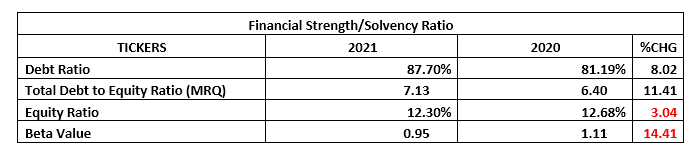Sources: Company Report, NGX, Investdata Research

Profitability Ratios

• Pre-Tax Margin rose by 17.56% from the corresponding period’s estimate of 14.34% at the end of 2020 first quarter.
• Interest Expense figure posted for the year is the same as 30.58% of the Estimated Gross Earnings figure; which is a 9.43% drop from the previous quarter’s estimate of 33.77%
• Return Achieved on Average Equity used through the quarter was estimated at 2.34% this is below the 2.37% return achieved in 2020 first quarter.
• See the table below for detailed profitability ratios and comparison: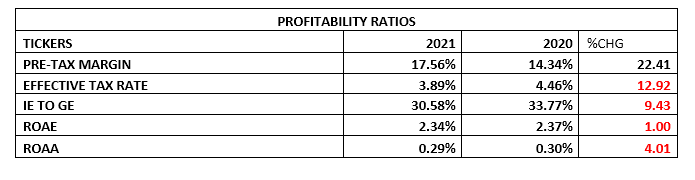Sources: Company Report, NGX, Investdata Research

Efficiency Ratios

• Operating Expenses is estimated at 41.93% of the Gross Earnings Value estimate for the period, this is an improvement in efficiency when compared to the 37.35% estimated from 2020 first quarter financial statistics.
• On the other hand, Gross Earnings was estimated at 1.71% of Total Assets, which is a 22.05% drop in efficiency, when compared to estimates from the corresponding quarter’s estimate.
• Total Loan to Deposit Ratio achieved at the end of the quarter is same as 63.54% up from the 62.76% achieved in 2020 first quarter. We note that this is slightly below the CBN mandated 65% for all financial institutions.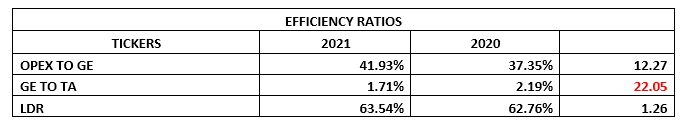Sources: Company Report, NGX, Investdata Research

Investment/Valuation Ratios

• Profit made on each unit of Union Bank shares at the end of the 2021 first quarter is estimated at N0.21 same as in the 2020 first quarter.
• Total Comprehensive Income per unit is also estimated at N0.03, versus N0.07 in corresponding quarter of 2020.
• Our adjusted PE/Ratio for first quarter stood at 5.75x as against 8.17x in the corresponding quarter.
• The said earnings per share is same as 4.35% of the current market price of Union Bank Plc shares on the floor of the exchange when the result was released.
• The current Book Value of Union Bank is N9.11, a 4.23% improvement over the N8.74 estimated in the comparable quarter of last year.
• Confirming the stock’s seemingly under-priced position is the Price to Book Value ratio that stood clearly below unity.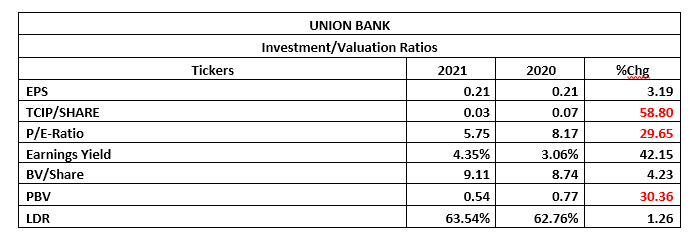Sources: Company Report, NGX, Investdata Research

Valuation

To achieve our fair valuation for Union Bank, we worked with the last few improved full-year earnings, but nevertheless, dividend growth remained static and has disadvantaged the upper valuation. Thus, we valued each unit of Union Bank shares at N3.78. Considering our value to the current market price on the floor of the exchange as at the time this report was compiled, we Rated it a Hold.

Check Also
Close